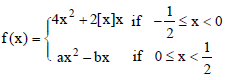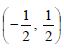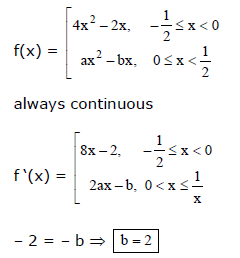# Letwhere [x] denotes the greatest integer function, thena)f(x) is continuous and differentiable infor all a, provided b =2b)f(x) is continuous and differentiable inif f(A) = 4, b = 2c)f(x) is continuous and differentiable inif a = 4 and b = 0d) for no choice of a and b, f(x) is differentiable inCorrect answer is option 'A'. Can you explain this answer? Related Test: Part Test- 7 (JEE Main 2018)

## JEE QuestionSher Singh Oct 23, 2020